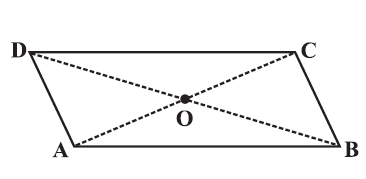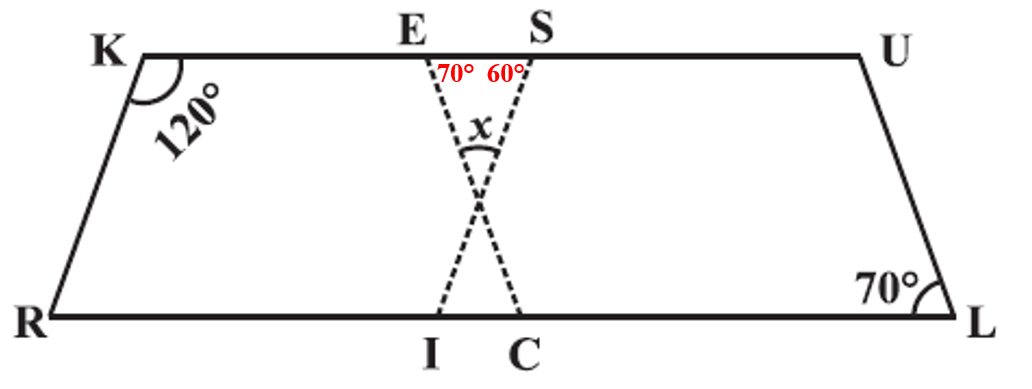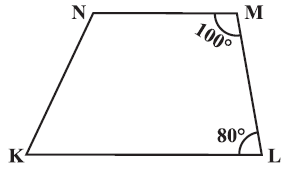# vs.eyeandcontacts.com

## Chapter 3 Understanding Quadrilaterals Exercise 3.3

Question 1: Given a parallelogram ABCD. Complete each statement along with the definition or property used.(ii) ∠DCB = ……
(iii) OC = ……
(iv) m ∠DAB + m ∠CDA = ……

(i) AD = BC (opposite sides are equal and parallel)
(ii) ∠DCB = ∠DAB (Opposite angles are equal)
(iii) OC = OA (Diagonals bisect each other)
(iv) m ∠DAB + m ∠CDA = 180° (Interior opposite angles, as AB||DC)

Question 2: Consider the following parallelograms. Find the values of the unknown x, y, zi)
y = 100° (opposite angles are equal in parallelogram)
y + x = 180° (adjacent angles are supplementary)
100 + x = 180°
x = 80°
x = z = 80° (opposite angles are equal in parallelogram)

ii)
50° + y = 180° (adjacent angles in a parallelogram are supplementary)
50° + x = 180° (adjacent angles in a parallelogram are supplementary)
y = 130°
x = 130°
y = z = 130° (interior alternate angles)

iii)
x = 90° (Vertically Opposite Angles)
y + x + 30° = 180° (angle sum property of a triangle)
y + 90° + 30° = 180°
y + 120° = 180°
y = 180 - 120
y = 60°
y = z = 60° (interior alternate angles)

iv)
y = 80° (opposite angles are equal)
x + 80° = 180° (adjacent angles are supplementary)
x = 100°
z = 80° (corresponding angles)

v)
y = 112° (opposite angles are equal)
y + 40° + x = 180° (angle sum property of a triangle)
112° + 40° + x = 180°
152° + x = 180°
x = z = 28° (interior alternate angles)

Question 3: Can a quadrilateral ABCD be a parallelogram if
(i) ∠D + ∠B = 180°?
(ii) AB = DC = 8 cm, AD = 4 cm and BC = 4.4 cm?
(iii)∠A = 70° and ∠C = 65°?

i) Yes, a quadrilateral ABCD be a parallelogram if ∠D + ∠B = 180°.
ii) No, in a parallelogram, opposite sides are equal, but AD ≠ BC.
iii) No, in a parallelogram, opposite angles are equal, but ∠A ≠ ∠C).

Question 4: Draw a rough figure of a quadrilateral that is not a parallelogram but has exactly two opposite angles of equal measure.Question 5: The measures of two adjacent angles of a parallelogram are in the ratio 3:2. Find the measure of each of the angles of the parallelogram.

Ratio of adjacent angles = 3:2
Find: Measure of each angle
(Adjacent angles of the parallelogram are supplementary )
3x + 2x = 180°
5x = 180°
x = 36°

3x = 108°
2x = 72°
Therefore, the angles of parallelogram are 72°, 108°, 72°, 108°.

Question 6: Two adjacent angles of a parallelogram have equal measure. Find the measure of each of the angles of the parallelogram.
Find: Measure of each angle
(Adjacent angles of parallelogram are supplementary)
x + x = 180°
2x = 180°
x = 90°
Therefore, the measure of each of the angle of the parallelogram is 90°.

Question 7: The adjacent figure HOPE is a parallelogram. Find the angle measures x, y and z. State the properties you use to find them.HOPE is a parallelogram.
y = 40° (alternate interior angles)
In triangle HPO,
70° = y + z (exterior angle property of a triangle)
z = 30°x = 110° (opposite angles are equal in parallelogram)
Therefore, x = 110°, y = 40°, z = 30°.

Question 8: The following figures GUNS and RUNS are parallelograms. Find x and y. (Lengths are in cm)i)
GUNS is a parallelogram.
Opposite sides are equal.
3x = 18
x = 18/3
x = 6

3y - 1 = 26
3y = 26 + 1
3y = 27
y = 27/3
y = 9
Therefore, x = 6, y = 9.

ii)
RUNS is a parallelogram.
Opposite diagonals are equal.
20 = y + 7
y = 20 - 7
y = 13

16 = x + y
16 = x + 13
x = 16 - 13
x = 3
Therefore, y = 13, x = 3.

Question 9: In the above figure both RISK and CLUE are parallelograms. Find the value of x.RISK parallelogram (adjacent angles are supplementary)
CLUE parallelogram (opposite angles are equal)Angle sum property of triangles
= 70° + 60° + x = 180°
= 130° + x = 180°
= x = 180 - 130°
= x = 50°
Therefore, x = 50°.

Question 10: Explain how this figure is a trapezium. Which of its two sides are parallel? (Fig 3.32)The co-interior angles ∠M + ∠L = 180°. The lines NM and KL are parallel. KLMN is a trapezium as one pair of opposite sides are parallel.

Question 11: Find m∠C in Fig 3.33 if AB || DC?Given
AB || DC
BC is a transversal.
Co-interior angles are supplementary.
m∠A + m∠C = 180°
120° + m∠C = 180°
∠C = 60°
Therefore, measure of ∠C is 60°.

Question 12: Find the measure of ∠P and ∠S if SP || RQ ? in Fig 3.34. (If you find m∠R, is there more than one method to find m∠P?)Given
SP || RQ
PQ and SR are transversal.
Co-interior angles are supplementary.
∠P + ∠Q = 180°
∠S + ∠R = 180°
∠P + 130° = 180°
∠P = 50°